# Inter Maths Solutions for Limits and Continuity Exercise 8(d)

intermediate maths solutions for Limits and Continuity exercise 8(d) textbook..

Thses are very easy to understand.

Observe the example problems and solutions given in the textbook.

Study the textbook lesson very well.

You can also see

Inter Maths 1A textbook solutions

Inter Maths 1B textbook solutions

Inter Maths IIA textbook solutions

Inter Maths IIB textbook solutions

Limits and Continuity

Exercise 8(a)

Exercise 8(b)

Exercise 8(c)

Exercise 8(d)

Exercise 8(e)

Nios maths 311 book 1 Sets 1.1 solutions

M

# Solutions for Limits and Continuity Inter

Chapter 8 – Limits and Continuity

Exercise 8(d)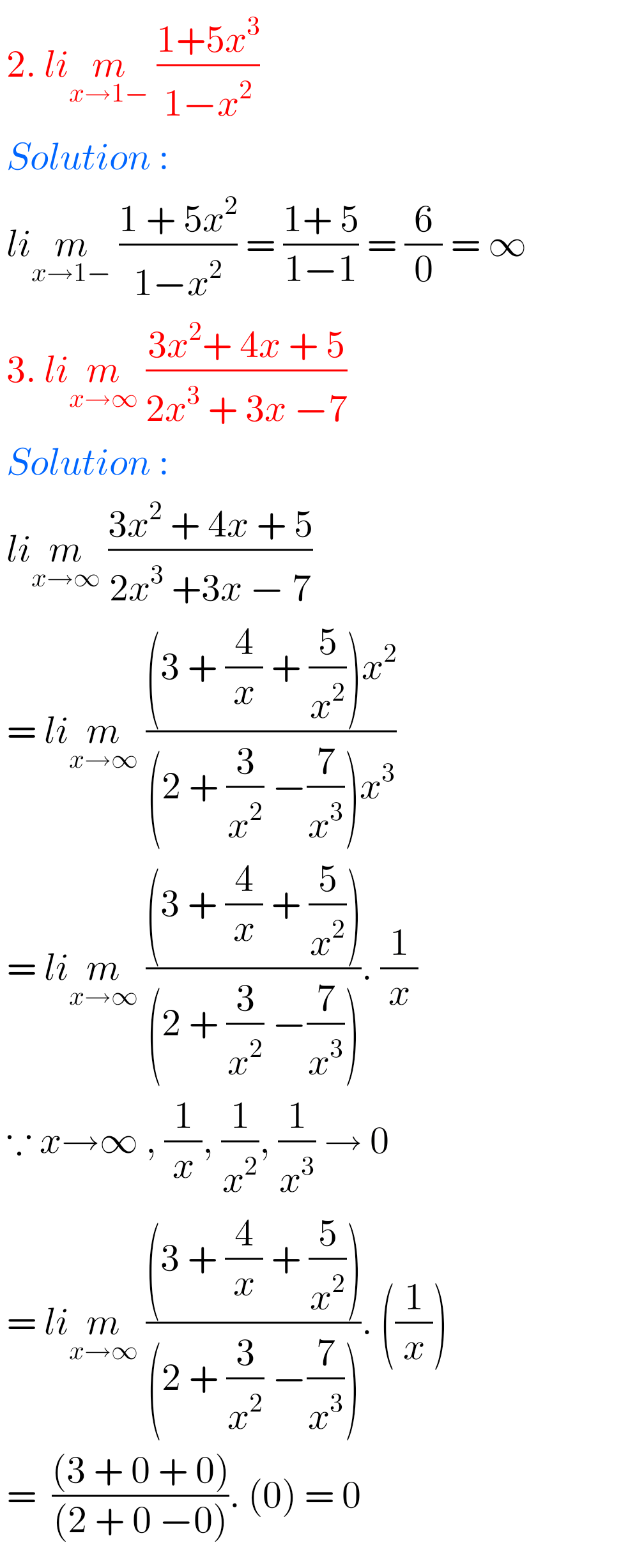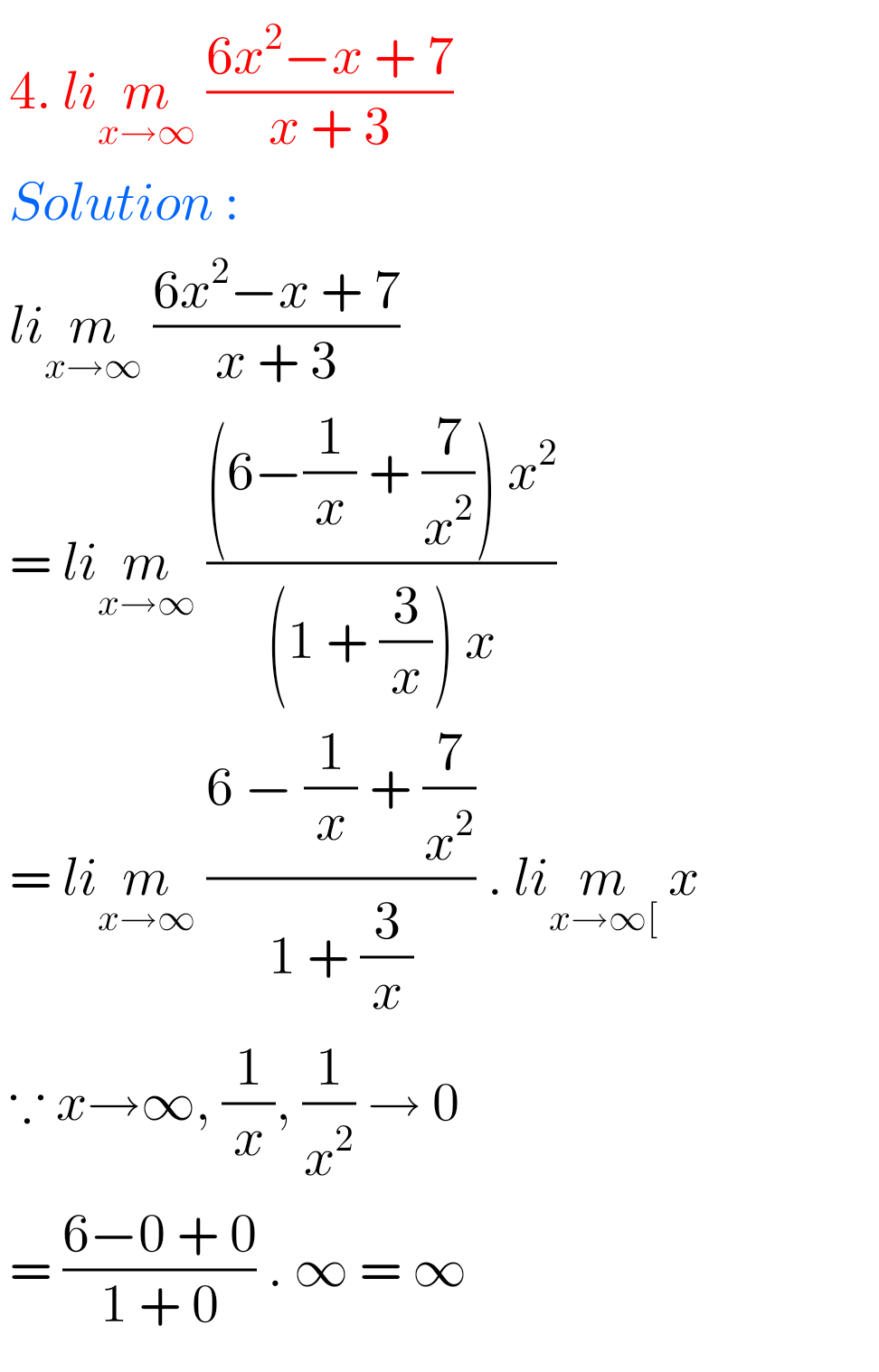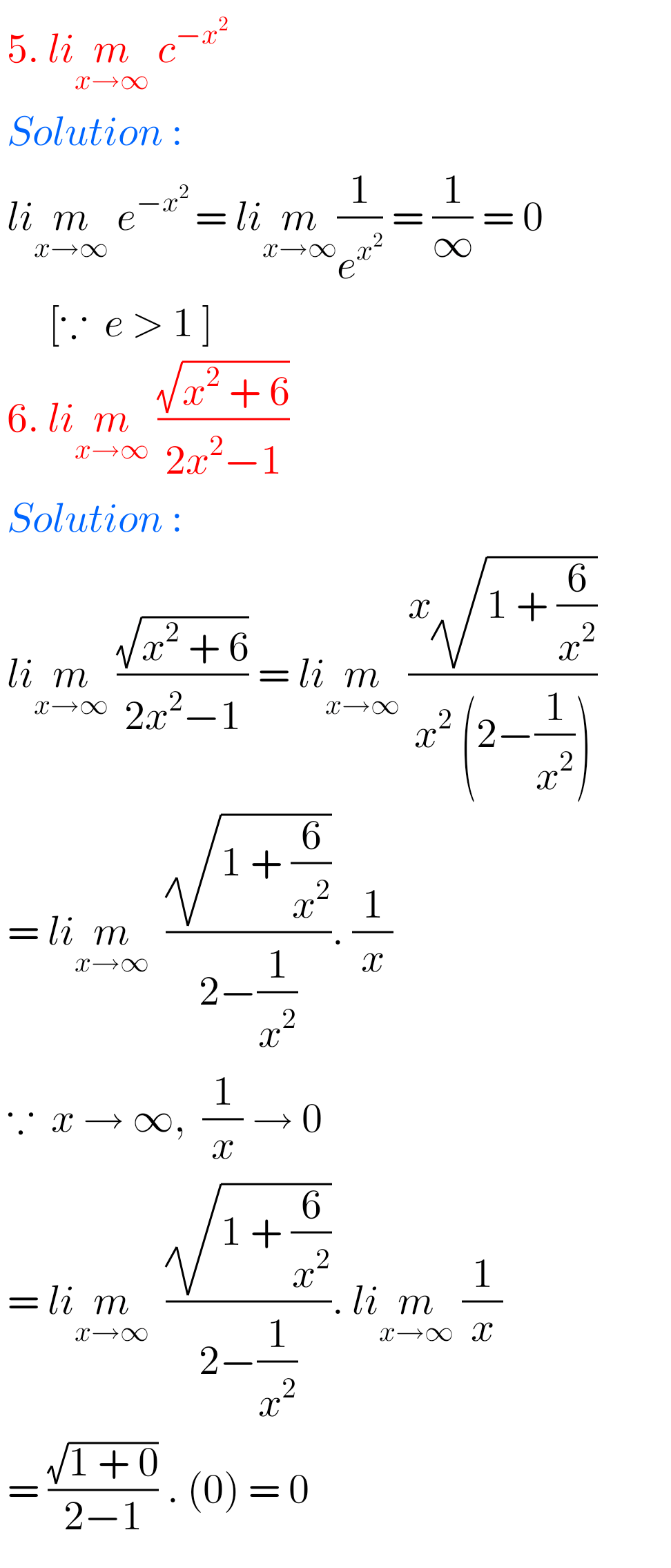## Maths solutions for exercise 8(d) Limits and Continuity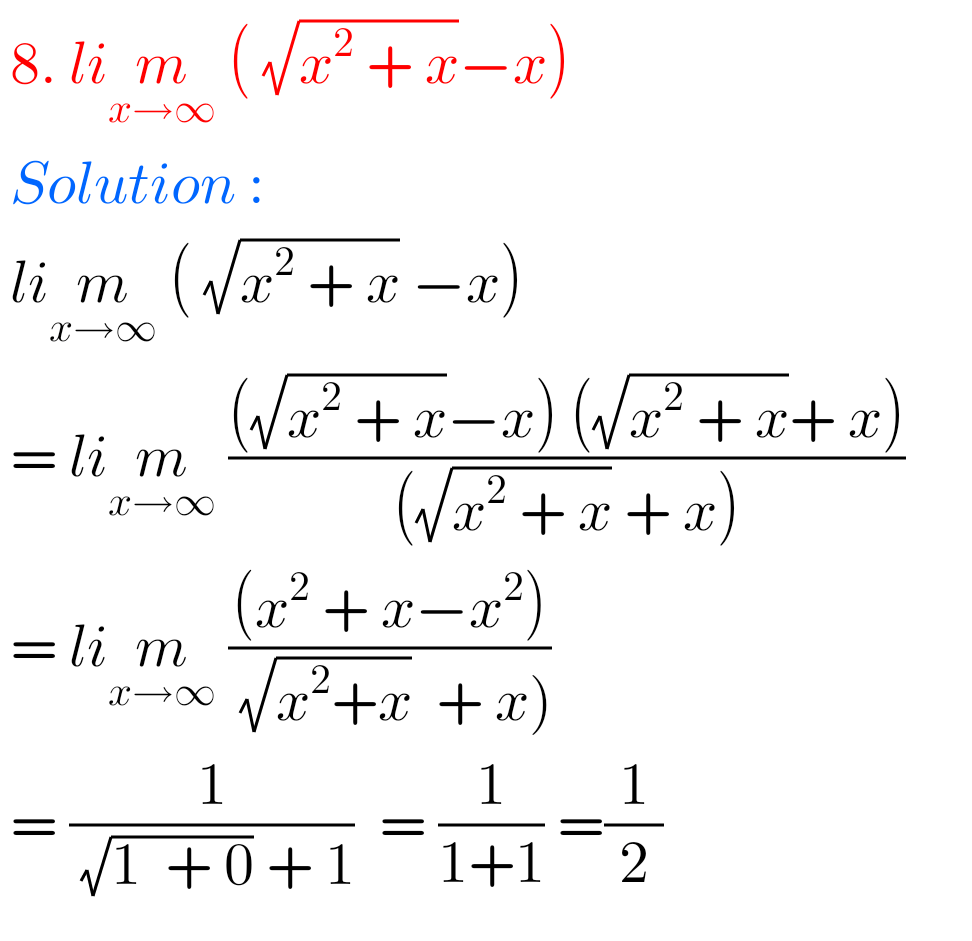M

### Exercise 8(d) Solutions Inter Limits and Continuity

M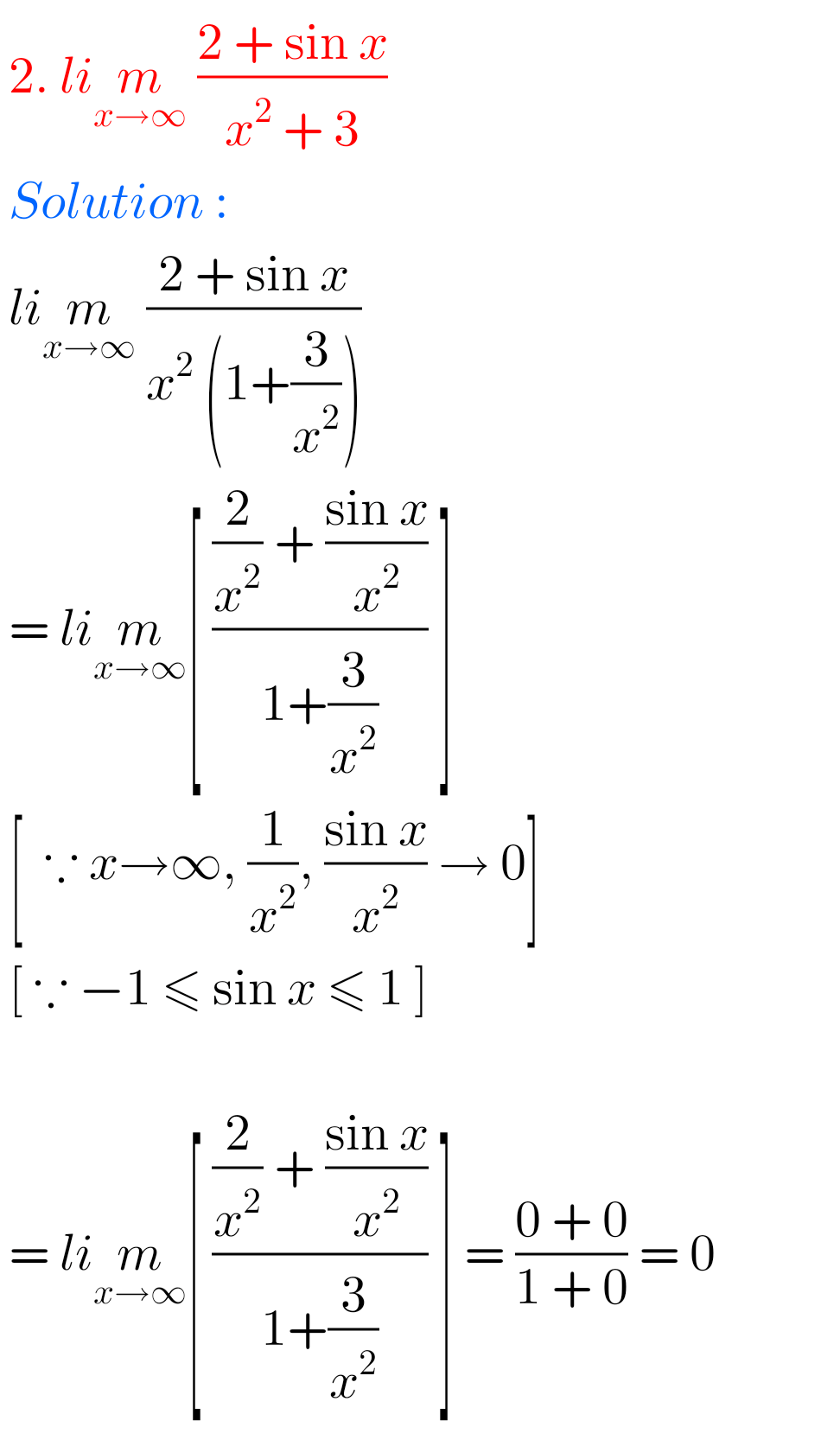Note : Observe the solutions and try them in your own method.

You can see the solutions for junior inter maths 1b solutions.

1. Locus

Straight lines sa

Straight lines la

11. Rate measure

SSC Maths solutions class 10

Ncert solutions for  Integers class 6

Ncert maths class 6 chapter 14 solutions

Nios maths 311 book 1 Sets 1.1 solutions